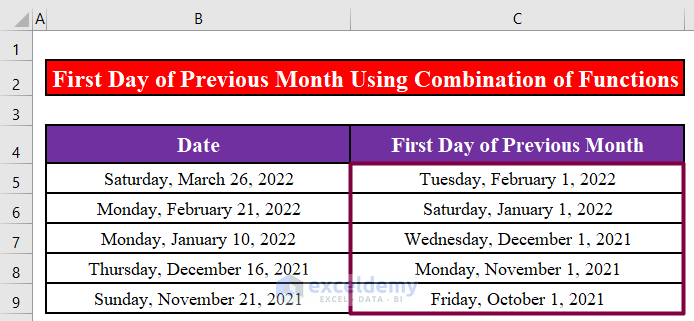# How to Calculate First Day of Previous Month in Excel (2 Methods)

Get FREE Advanced Excel Exercises with Solutions!

If you are working with dates in Excel, you might need to find out the first day of the month before the current month you are working with. In Excel, you can calculate the first day of the previous month with some very simple functions like EOMONTH, DATE, YEAR, and MONTH. In this tutorial, I will show you how to calculate first day of previous month in Excel.

## How Calculate First Day of Previous Month in Excel: 2 Easy Ways

The image below shows an Excel worksheet where we have some dates in the Date column and we have calculated the first days of the months before the months of the respective dates in the First Day of Previous Month column. We will use the EOMONTH, DATE, YEAR, and MONTH functions in Excel to calculate the first date of the previous month.### Method 1: Use the EOMONTH Function to Calculate the First Day of the Previous Month

Step 1:

• First, we will write down the following formula in cell C5.
`=EOMONTH(B5,-2)+1`Formula Breakdown:

• The EOMONTH function returns the serial number for the month’s last day, which is the specified number of months before or after the start date.
• Upon pressing ENTER, we will see that the formula returns the first day of the last month for the date in cell B5.Step 2:

• Now, we will drag the fill handle of cell C5 downward to apply the formula to the rest of the cells.• Finally, we will see that the formula returns the first day of each previous month for the dates in the Date.### Method 2: Calculate the First Day of the Previous Month Combining the DATE, YEAR, and MONTH Functions in Excel

Step 1:

• First, we will write down the following formula in cell C5.
`=DATE(YEAR(B5),MONTH(B5)-1,1)`Formula Breakdown:

• The DATE function returns the date with the given year, month, and day.
• The YEAR function returns the year corresponding to a date.
• The MONTH function returns the month of a date represented by a serial number.
• Upon pressing ENTER, we will see that the formula returns the first day of the last month for the date in cell B5.Step 2:

• Now, we will drag the fill handle of cell C5 downward to apply the formula to the rest of the cells.
• Finally, we will see that the formula returns the first day of each previous month for the dates in the Date.

##• We can also use the same formula with the TODAY() function in Excel to find out the first day of the month preceding the current month. We will write down the following formula in cell C5.
`=DATE(YEAR(TODAY()),MONTH(TODAY())-1,1)`• Upon pressing ENTER, we will see that the formula returns the first day of the month preceding the current month.### Bonus: Merge the DATE, YEAR, and MONTH Functions to Calculate the Last Day of the Previous Month in Excel

We have also added a bonus method for you! Using this method you can easily calculate the last day of the previous month. Let’s see how it’s done.

Step 1:

• First, we will write down the following formula in cell C5.
`=DATE(YEAR(B5),MONTH(B5),0)`• Upon pressing ENTER, we will see that the formula returns the last day of the previous month for the date in cell B5.Step 2:

• Now, we will drag the fill handle of cell C5 downward to apply the formula to the rest of the cells.• Finally, we will see that the formula returns the last day of each previous month for the dates in the Date.Read More: Excel Formula for Current Month and Year

## What is ExcelDemy?

ExcelDemy Learn Excel & Excel Solutions Center provides free Excel tutorials, free support , online Excel training and Excel consultancy services for Excel professionals and businesses. Feel free to contact us with your Excel problems.ASM Arman

Hi there! I am ASM Arman. I Completed B.Sc. in Naval Architecture and Marine Engineering. I take a great interest in learning about new technologies and sharing my ideas and thoughts on them with others. Please do reach out to me if you have any queries or recommendations. Have a great day!!!

We will be happy to hear your thoughtsAdvanced Excel Exercises with Solutions PDF